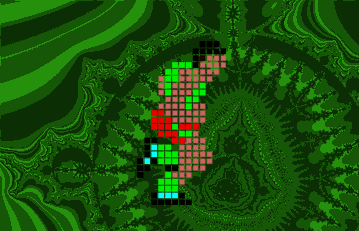8.1 - Random Walk on a FractalHandsOn Activities:

30. Random Walk on a Fractal

The length of one step in Figure 8.2 as the distance from point A to point B. Then the distance from point A to point B'' is four steps. (From now on, we will call each intersection-where two or more lines meet-a point or a grid point.) What do we mean by a random walk on the gasket? If we are at point A or at either of the two point marked B'' in Figure 8.2(b), then we are connected to only two other points on the gasket. A random step from A or B'' will take us to one of the two nearest grid points, with a 0.5 probability of arriving at each point. If we are at any other point on the gasket, then we are connected to four other grid points. Then a random step will take us to one of these four nearest grid points, with a 0.25 probability of arriving at each point, just as in the case of the random walk on a square grid.

 Q8.3: Speculate: If we start a random walker at Point A, how many steps will it take on average for it to reach Point B''? The points are separated by a distance of four steps. If this were a square grid, we might guess that the average number of steps necessary to travel this distance would be 16. For an explanation, see Unit 3.5.

 Q8.4: Do you think that it will take on average a greater or lesser number of steps than 16 to go from Point A to Point B''? Write down a brief argument to explain your prediction.

Previous: Unit 8 - Motion on a Fractal